# PSEB 5th Class Maths Solutions Chapter 1 Numbers Ex 1.1

Punjab State Board PSEB 5th Class Maths Book Solutions Chapter 1 Numbers Ex 1.1 Textbook Exercise Questions and Answers.

## PSEB Solutions for Class 5 Maths Chapter 1 Numbers Ex 1.1

Question 1.
Read the abacus and write the numbers:
(a)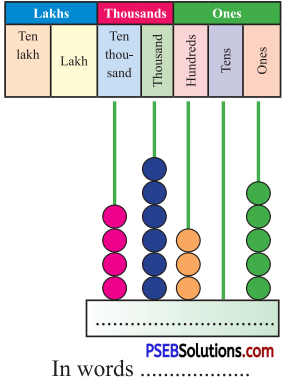Solution:
In words: Forty six thousand three hundred five

(b)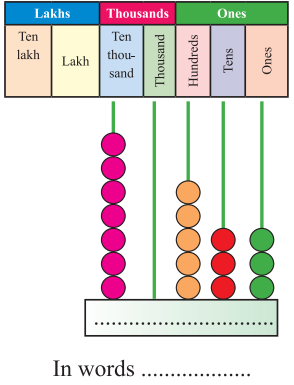Solution:
In words: Seventy thousand five hundred thirty three

(c)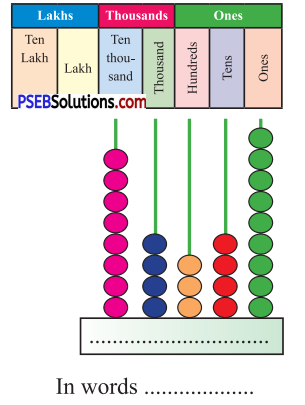Solution:
In words: Eighty four thousand three hundred forty nine

(d)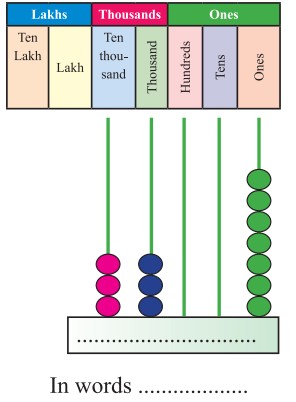Solution:
In words: Thirty three thousand seven.

Question 2.
Write the following numbers using place value chart:
(a) 3868
(b) 17605
(c) 41123
(d) 92856
(e) 20203
(f) 99728.
Solution: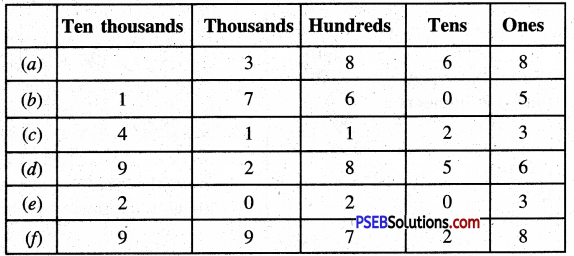Question 3.
Write in words:
(a) 2462
(b) 8988
(c) 19050
(d) 33006
(e) 20198
(f) 59045
(g) 68390.
Solution:
(a) 2462 = Two thousand four hundred sixty two
(b) 8988 = Eight thousand nine hundred eighty eight
(e) 19050 = Nineteen thousand fifty
(d) 33006 = Thirty three thousand six
(e) 20198 = Twenty thousand one hundred ninety eight
(f) 59045 = Fifty nine thousand fourty five
(g) 68390 = Sixty eight thousand three hundred ninety.

Question 4.
Write the following numbers in figures:
(a) One thousand seven hundred forty five.
(b) Thirty three thousand eight hundred seventy five.
(c) Seventy seven thousand seventy seven.
(d) Fifty thousand five hundred five.
(e) Ninety thousand eight hundred six.
(f) Eighty thousand eight hundred eighty.
(g) One lakh.
Solution:
(a) 1745
(b) 33875
(c) 77077
(d) 50505
(e) 90806
(f) 80880
(g) 100000.

Question 5.
Write the successor of the following numbers :
(a) 998
(b) 10000
(c) 2018
(d) 99999
(e) 48675
(f) 40009.
Solution:
(a) Successor of 998 = 998 + 1
= 999
(b) Successor of 10000 = 10000+ 1
= 10001
(c) Successor of 2018 = 2018 + 1
= 2019
(d) Successor of 99999 = 99999 + 1
= 100000
(e) Successor of 48675 = 48675 +1
= 48676
(f) Successor of 40009 = 40009 + 1
= 40010Question 6.
Write the predecessor of the following numbers :
(a) 24855
(b) 99999
(c) 39999
(d) 79890
(e) 50000
(f) 23456.
Solution:
(a) Predecessor of 24855 = 24855 – 1
= 24854
(b) Predecessor of 99999 = 99999 – 1
= 99998
(c) Predecessor of 39999 = 39999 – 1
= 39998
(d) Predecessor of 79890 = 79890 – 1
= 79889
(e) Predecessor of 50000 = 50000- 1
= 49999
(f) Predecessor of 23456 = 23456 – 1
= 23455